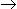# Chemical Engineering - Chemical Reaction Engineering

### Exercise :: Chemical Reaction Engineering - Section 2

46.

The catalyst in a second order reversible reaction increases the rate of the forward reaction

 A. and decreases that of backward reaction. B. and backward reaction equally. C. only. D. to a greater extent than that of the backward reaction.

Explanation:

No answer description available for this question. Let us discuss.

47.

Catalyst carriers

 A. have very high selectivity. B. increase the activity of a catalyst. C. provide large surface area with a small amount of active material. D. inhibit catalyst poisoning.

Explanation:

No answer description available for this question. Let us discuss.

48.

For the liquid phase zero order irreversible reaction AB, the conversion of A in a CSTR is found to be 0.3 at a space velocity of 0.1min-1 . What will be the conversion for a PFR with a space velocity of 0.2 min-1? Assume that all the other operating conditions are the same for CSTR and PFR.

 A. 0.15 B. 0.3 C. 0.6 D. 0.9

Explanation:

No answer description available for this question. Let us discuss.

49.

A high space velocity means that a given

 A. reaction can be accomplished with small reactor. B. conversion can be obtained with a high feed rate. C. both (a) and (b). D. none of these.

Explanation:

No answer description available for this question. Let us discuss.

50.

In flow reactors, the performance equations interrelate the rate of reaction to the

 A. feed rate. B. reactor volume, C. extent of reaction. D. all (a), (b) and (c).